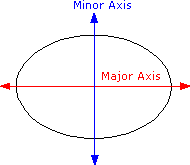## How to Calculate and Solve for the Perimeter of an Ellipse | Nickzom CalculatorThe image above is an ellipse.

To compute the perimeter of an ellipse, two essential parameters are needed and this parameters are axis (a) and axis (b).

The formula for calculating the perimeter of an ellipse:

P = π [3(a + b) – √((3a + b)(a + 3b))]

Where;

P = Perimeter of the ellipse
a = Axis of the ellipse
b = Axis of the ellipse

Let’s solve an example;
Given that the axis of the ellipse (a) is 19 cm and axis of the ellipse (b) is 31 cm. Find the perimeter of the ellipse?

This implies that;
a = Axis of the ellipse = 19 cm
b = Axis of the ellipse = 31 cm

P = π [3(a + b) – √((3a + b)(a + 3b))]
P = 3.142 [3(19 + 31) – √((3 x 19 + 31)(19 + 3 x 31))]
P = 3.142 [3(50) – √((57+ 31)(19 + 93))]
P = 3.142 [150 – √((88)(112))]
P = 3.142 [150 – √(9856)]
P = 3.142 [150 – 99.28]
P = 3.142 [50.72]
P = 3.142 x 50.72
P = 159.26

Therefore, the perimeter of the ellipse is 159.26 cm.